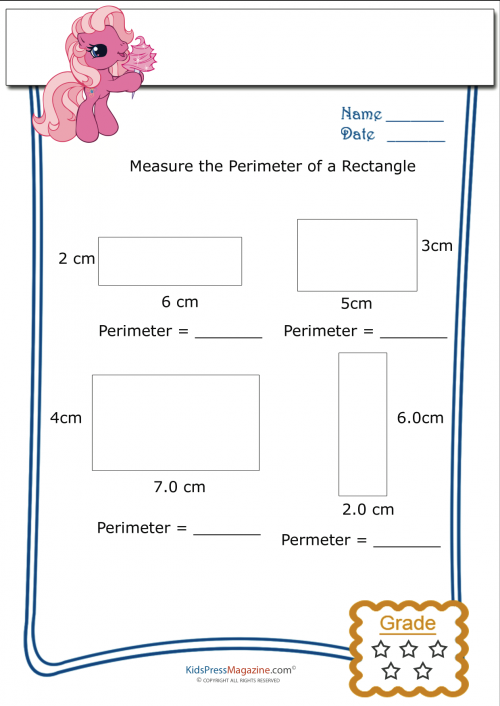# Measure Perimeter Worksheet – Rectangle 5

The easiest way to find the perimeter if a rectangle is to add together all four sides. However, the height of a rectangle is the same on each side; same with the width, it is the same top to bottom. Since we know this you can teach your children to easily calculate the perimeter by measuring just the height and width, doubling them and adding those two numbers together.

(h X 2) + (w X 2) = Perimeter